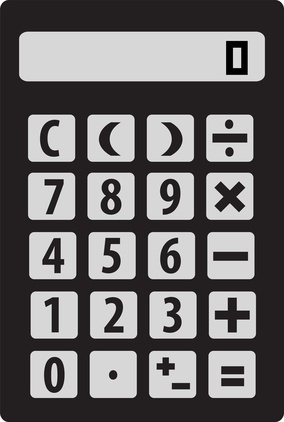• - as a whole number like "3" or "26"
• - as a decimal number like "7.5" or "16.2"
• - as a fraction like "1/2" or "3 1/3"### Conversion Inches » mm

⇓⇑ equal to⇓⇑ equal to

### Correlation between Inch and mm

As maybe already recognized, one Inch is similar to 2.54 centimeter. Centimeter is always a decimal multiple of the unit meter. Also Millimeter is part of the metric system and therefore also a decimal multiple of the unit meter. (exactly 10-2 from centimeter to meter and 10-3 from millimeter to meter). Therefore it's always possible to easily convert between centimeter and millimeter. Due to the difference of 10-1 it can be said that one centimeter is always the same then 10 millimeter. Due to this conclusion we can say that one Inch is comparable to exactly 25.4 Millimeter.

### Conversion from Inches to mm

The conversion from Inch or Inches to millimeter is nowadays still usual and necessary in many areas. Of course for a lot of parts like monitor screens for TVs, computers or smartphones the declaration is in Inches due to the bigger size. But for a lot of stuff for industry or craft the size is often used in millimeter because the parts are so small. For example for a screw often the size in millimeter is used. Also for threads or for pipes the indications are often made in millimeter. So when you know that there is quite no difference between millimeter and centimeter it's not hard to instantly convert.

### Inches, millimeter and centimeter compared

The formula for the conversion from Inches to mm is quietly the same than for the conversion from Inches to cm. You can always keep in mind that one centimeter is exactly the same than 10 millimeter. So as you already know one Inch is the same than 2.54 centimeter. If you want to get the number in millimeter you can just shift the point one digit to the right. So in this case it can be said that one Inch is completely the same than 25.4 millimeter.
1 Centimeter = 10 Millimeter
1 Inch = 2.54 Centimeter (cm)
1 Inch = 25.4 Millimeter (mm)